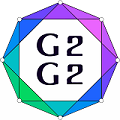#Graphs and Groups, Geometries and GAP (G2G2) Summer School - External Satellite Conference of 8ECM

27 June 2021 to 3 July 2021
Rogla, Slovenia
UTC timezone

## Type IV-II codes over $\mathbb{Z}_4$ constructed from generalized bent functions

Not scheduled
20m
Rogla, Slovenia

#### Rogla, Slovenia

Hotel Planja, Rogla 1, 3214 Zreče, Slovenia
Oral presentation

### Speaker

Dr Sara Ban (Department of Mathematics, University of Rijeka)

### Description

A Type IV-II $\mathbb{Z}_4$-code is a self-dual code over $\mathbb{Z}_4$ with the property that all Euclidean weights are divisible by eight and all codewords have even Hamming weight.
The subject of this talk is a construction of Type IV-II codes over $\mathbb{Z}_4$ from generalized bent functions.

We use generalized bent functions for a construction of self-orthogonal codes over $\mathbb{Z}_4$ of length $2^m$, for $m$ odd, $m\geq3$, and prove that for $m\geq5$ those codes can be extended to Type IV-II $\mathbb{Z}_4$-codes. From that family of Type IV-II $\mathbb{Z}_4$-codes, we construct a family of self-dual Type II binary codes by using the Gray map.

We also consider the weight distributions of the obtained codes and the structure of the supports of the minimum weight codewords, which we use for a construction of 1-designs.
Some of the constructed 1-designs are affine resolvable 1-designs.
For the constructed 1-designs, we examine the properties of the corresponding block intersection graphs and obtain strongly regular graphs in two cases.

### Primary authors

Dr Sara Ban (Department of Mathematics, University of Rijeka) Prof. Sanja Rukavina (Department of Mathematics, University of Rijeka)